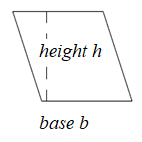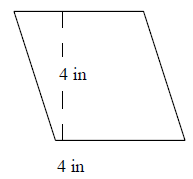# Area of a parallelogramA parallelogram has two pairs of parallel equal opposite sides. It has a base and a height which is the perpendicular distance between the base and its opposite parallel side.

Area of a parallelogram

Area of a parallelogram is found by taking a triangle and relocating it such that parallelogram becomes a rectangle whose area = l × w

Effectively the length l is the base b of the parallelogram and width w is the height h of the parallelogram.

Formula to find area of a parallelogram

Area of parallelogram is = base × height = b × h

Find the area of the following parallelogram.### Solution

Step 1:

Area of Parallelogram = b × h; b = base = 4 in; h = height = 42 in.

Step 2:

Area of given parallelogram = 4 × 42 = 168 square in.

Find the area of the following parallelogram.### Solution

Step 1:

Area of Parallelogram = b × h; b = base = 4 in; h = height = 4 in.

Step 2:

Area of given parallelogram = 4 × 4 = 16 square in.